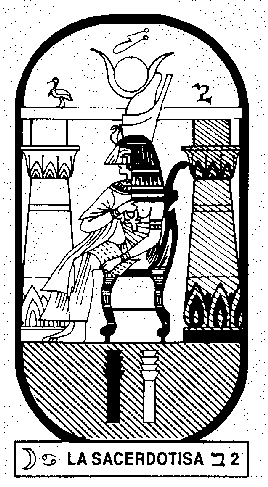# what is the inner urgency and what is useful for to know it?

### Answers from the books of Samael Aun WeorTHE INNER URGENCY
The Inner Urgency is calculated by making the kabalistic addition of the date of birth, the
day, the month and the year.

EXAMPLE 1
Victor Manuel Chavez: 17 November 1921.

Day 17 = 1 + 7 = 8
Month 11 = 1 + 1 = 2
Year 1921 = 1 + 9 + 2 + 1 = 13 = 1 + 3 = 4
Day 8
Month 2
Year 4
14 = 1 + 4 = 5

5: Arcanum 5 of the Tarot is rigor, study, science, analysis, investigation, intellect. That
Inner Urgency makes him a reasoning person.

EXAMPLE 2
Mr Rodriguez: 5 June 1905.

Day 5
Month 6
Year 1905 = 1+ 9 + 0 + 5 = 15 => 1 + 5 = 6
Day 5
Month 6
Year 6
17 = 1 + 7 = 8

The 8 makes us patient, passive, energetic, just, upright. The person has had sufferings,
struggles, study, has had to pass through many tests.

EXAMPLE 3
Eduardo de Portillo: 5 July 1932.

Day 5
Month 7
Year 1932 =1 + 9 + 3 + 2 = 15 => 1 + 5 = 6
Day 5
Month 7
Year 6
6 + 7 + 5 = 18 => 8 + 1 = 9

9: Ninth Sphere. The Hermit in the midst of all, seeking the light, will achieve the
realisation of great genial works. The development of a tremendous energy has made him
a Hermit upon the path of existence.